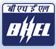# Placement Papers - BHELBHEL Mechanical Engineering Paper
Posted by :
Anannya
(314)
BHEL Mechanical Engineering Paper

I attended the BHEL Engineer Trainee written test in Mechanical stream. They conducted for four brances in Engineering: Comp sci, ECE, EEE and Mechanical. In Mechanical Branch there were a total of 240 questions. 120- Technical questions in Mechanical Engineering. 120- General Apptitude questions. (Quantitative Aptitude, Reasoning Ability, Logical thinking, English usage).

The questions were mainly asked in

Thermodynamics
Engineering   Mechanics
Theory   of machines
Production   tech
If you are thorough with your subject, you can really do well in this session.

[One Mark for correct answer, 1/5th for wrong answer. Five choices were given] Some questions which i remember are :

1)Unit of Entropy (J/kg K or kJ/kg K)?

2)A mass of 100kg is falling from a height of 1 m and penetrates the sand into for 1 m. What is the resistance force given by sand?

3)Ratio of specific heats of air ? (1.41)

4)A body weighs 3 kg in air. If it is submerged in a liquid, it weighs 2.5 kg. What is the specific gravity of the liquid ?

5)Two cars travell in same direction at 40 km/hr at a regular distance. A car comes in a opposite direction in 60km/hr. It meets each car in a gap of 8 seconds. What is the distance between them?

6)A simple problem involing in hoops stress. For sphere: M= [3/2]*p*V* [density of pressure vessel material/Maximum working stress it can tolerate] For Thin walled pressure walls: Hoops stress or stress in the radial direction =p*r/2t?

7) A problem in force calculation in a body moving in a inclined surface?

8)Composition of bronze?
ans. Copper+zinc.

9) Compostion of stainless steel.?
ans. iron+chromium+nickel+carbon.

10) CI is manifactured in which process?
ans. cupola process

11)What percentage of carbon is preset in pig iron?
ans. 4.5 to 6%.

12)Water is available at 10m height. What is the pressure available?
ans= pressure=density*g*height;
p=1000*9.81*10;
p=98100N/m2.

13)What will happen if the speed of the centrifugal pump doubles?

14)The unit eV is widely used in ?
ans. Nuclear and atomic physics.

15)What will happen to the resistance, when the diameter of the conductor is doubled?

16)The power comsumed by a electrical device is 1000W at 250V, What is the resistance in the device?
ans. Power= Voltage*current,
Current=Power/Voltage,
Current,I=1000/250=4
amps. V=IR,
Therefore R=250/4=62.5 ohms.

17)Why DC current is not used in transformer?

18)On what principle the sonar/ radar works?

19)Bending moment diagram for the UDL is in what shape?

20)Function of the distributor in petrol vehicles?
ans. Spark timing.

21)Which is not present in CI engines?
ans. carburrettor.

22)What will happen if one cylinder recieves more amount of fuel spray from injectors than other injectors?

23)Purpose of draft tube in hydraulic turbines?

ans. The purpose of a draft tube is toconvert some of the kinetic energy of the flow from the runner (the rotating part of the turbine) intopressure energy and thereby increase the efficiency ofthe hydro power turbine.

24)What is the effect or reheater in the gas turbine?

The advantage of reheater is significantly increased thrust; the
disadvantage is it has very high fuel consumption and inefficiency.

25)Problems involving with fricition coefficient.

26)Factor of safety = Yeild stress/Working stress.

27)Which is the example of non parallel power transmission (Universal coupling)?

28)For perpendicular shafts worm gear is used?

29)A planet gear with 25 teeth is meshed with a sun gear of 100 teeth. Both are connected using a arm. How many rotations are needed for planet gear to complete one rotation around the sun gear?

30)What is equivalent spring constant for spring in parallel?

31) Some questions was asked related to boundary layer and vortex flow.

32) 5 questions were asked in PERT, Process planning, Product planning, Break even analysis.

33)How are tungsten and sintered composite materials are machined?
(Electro Discharge Machining, EDM)

General Apptitude:

very easy.

There are 120 questions .

English:

Detect the words with wrong spellings (5 questions).
Fill in the blanks with appropriate words(5 questions).
Two reading comprehensions were given(10 questions).
Find the error in the sentence (8 questions).

Reasoning:

Seating arrangement problems.
Find the relation between them.

Quantitative ability:

50% of the questions are from basic geometry .
Remaining were very general and easy. But many questions are there that\'s y you should consider time factor and be quick.